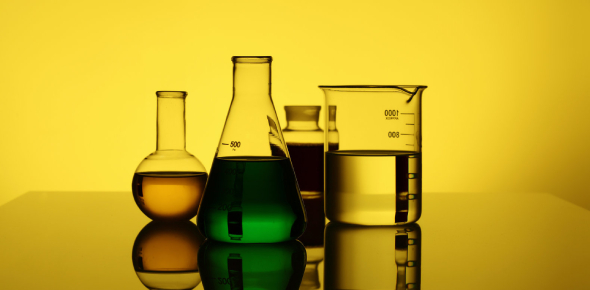# Take This Quiz On Introduction To Chemistry!

10 Questions | Total Attempts: 3047SettingsChemistry is the scientific study of matter which includes matter formation, composition, various attributes and the energy they consume or release through these transformations. It incorporates the science of biology and physics. This quiz has been created to test your basic concepts and knowledge about chemistry and chemical reactions. So, let's try out the quiz. All the best!

• 1.
Which of the following is not a part of the scientific method?
• A.

Experimenting

• B.

Observing

• C.

Proving

• D.

Hypothesizing

• 2.
Which of these steps of the scientific method involves stating a relationship but not proposing an explanation for the relationship?
• A.

Observation

• B.

Scientific law

• C.

Theory

• D.

Hypothesis

• 3.
Three states of matter.
• A.

Density, volume and weight

• B.

Solid, liquid, and gas

• C.

Water, metal and gases

• D.

All of the above

• 4.
A liquid has:
• A.

A definite shape and volume

• B.

A definite volume, but not a definite shape

• C.

A definite shape, but not a definite volume

• D.

A variable shape and volume

• 5.
Which of the following is a list of compounds?
• A.

Water, carbon dioxide, benzene

• B.

Gold, silver, brass

• C.

Brick, sand, concrete

• D.

Hydrogen, nitrogen, oxygen

• 6.
The temperature at which a substance changes from a liquid to a gas
• A.

Freezing point

• B.

Melting point

• C.

Boiling point

• D.

Condensation point

• 7.
Anything that has a definite volume but no definite shape
• A.

Solid

• B.

Liquid

• C.

Gas

• D.

All of the above

• 8.
Density is a measure of how much mass is contained in a given unit volume
• A.

True

• B.

False

• 9.
The amount of space something takes up.
• A.

Density

• B.

Volume

• C.

Mass

• D.

Force

• 10.
The temperature at which a substance changes from a solid to a liquid.
• A.

Condensation point

• B.

Boiling point

• C.

Freezing point

• D.

Melting point

Related TopicsBack to top